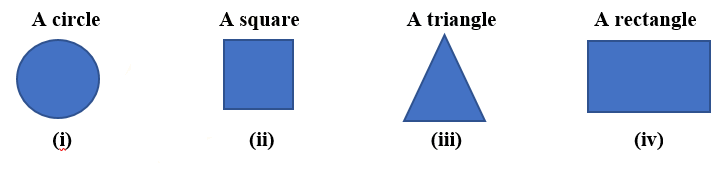# Ex.15.4 Q2 Visualising Solid Shapes - NCERT Maths Class 7

Go back to  'Ex.15.4'

## Question

Here are the shadows of some $$3-D$$ objects, when seen under the lamp of an overhead projector. Identify the solid(s) that match each shadow. (There may be multiple answers for these!)Video Solution
Visualizing Solid Shapes
Ex 15.4 | Question 2

## Text Solution

What is known?

What is unknown?

Reasoning:

A spherical object gives a circular shape shadow.

A cylindrical object gives a shadow of a line.

A cubical object gives a square shape shadow.

A cuboidal object gives a rectangular shape shadow.

A conical object gives a triangular shadow.

Steps:

Solids will be:

(i) A ball or sphere will give shadow of a circle.

(ii) A cube shape object like dice will give shadow of a square.

(iii) An ice- cream cone or a birthday cap will give the shadow of triangle.

(iv) A book or a cuboid shape object will give shadow of a rectangle.

Learn from the best math teachers and top your exams

• Live one on one classroom and doubt clearing
• Practice worksheets in and after class for conceptual clarity
• Personalized curriculum to keep up with school